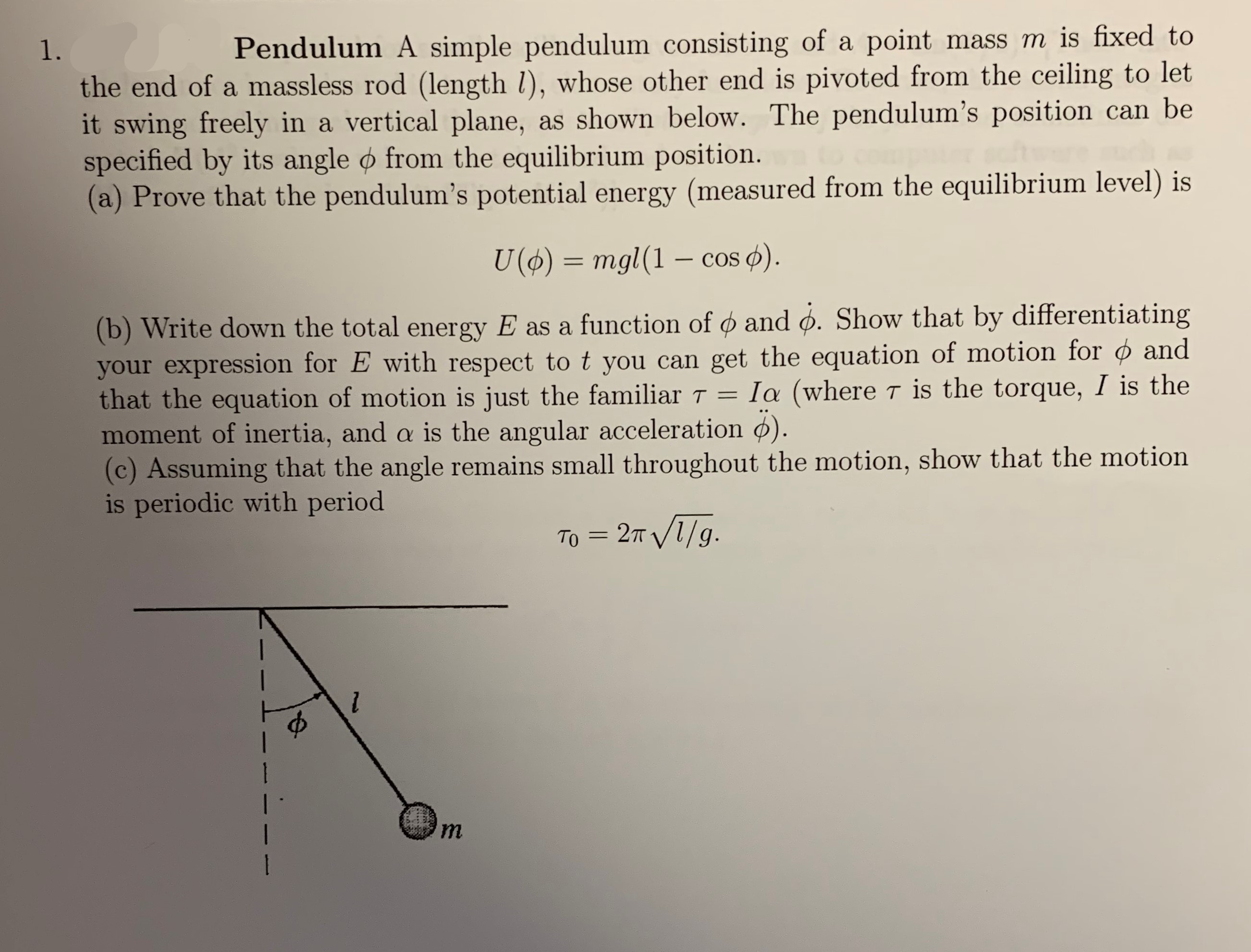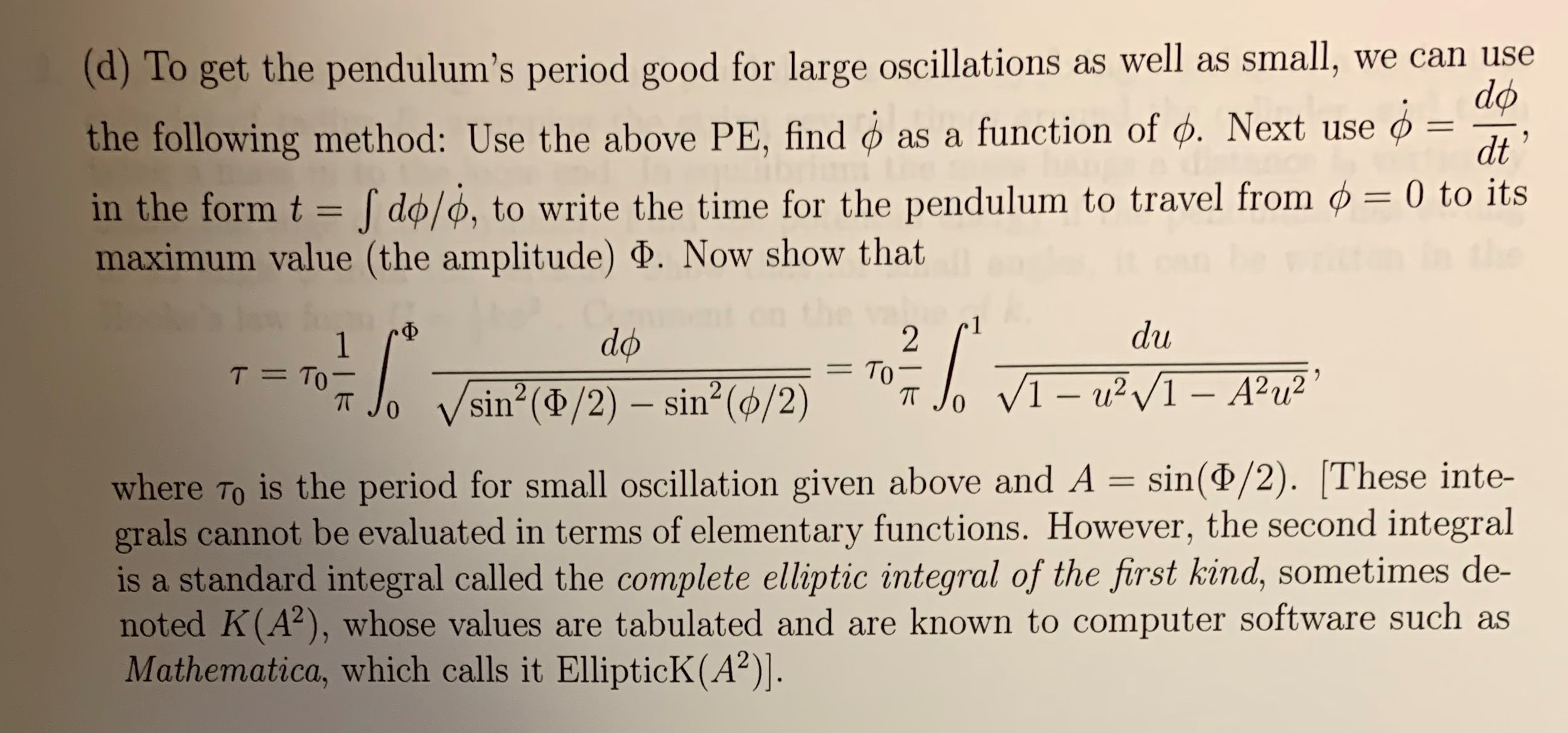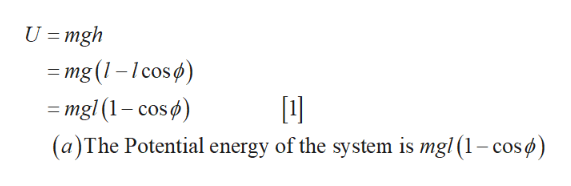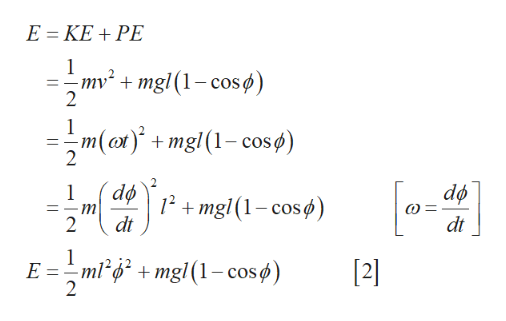# mass m is fixed toPendulum A simple pendulum consisting of a point1.the end of a massless rod (length /), whose other end is pivoted from the ceiling to letit swing freely in a vertical plane,specified by its angle o from the equilibrium position.(a) Prove that the pendulum's potential energy (measured from the equilibrium level) isas shown below. The pendulum's position can beU ()mgl (1 - cos )(b) Write down the total energy E as a function of and o. Show that by differentiatingyour expression for E with respect to t you can get the equation of motion for ¢ andthat the equation of motion is just the familiar Tmoment of inertia, and a is the angular acceleration ).(c) Assuming that the angle remains small throughout the motion, show that the motionis periodic with periodIa (where T is the torque, I is the- 2т V1/9.Tоm1 (d)To get the pendulum's period good for large oscillations as well as small,the following method: Use the above PE, findwe can usedoas a function of d. Next use11dtin the form tdo/o, to write the time for the pendulum to travel from ø = 0 to itsmaximum value (the amplitude) . Now show thatdu-Фdo2То -T TO1VI-u2I-A2u2sin (d/2) sin2 (ø/2)0is the period for small oscillation given above and A = sin(P/2). These inte-where Tograls cannot be evaluated in terms of elementary functions. However, the second integralis a standard integral called the complete elliptic integral of the first kind, sometimes de-noted K(A2), whose values are tabulated and are known to computer software such asMathematica, which calls it EllipticK (A2)].

Question
57 views

can you do part d pleasehelp_outlineImage Transcriptionclosemass m is fixed to Pendulum A simple pendulum consisting of a point 1. the end of a massless rod (length /), whose other end is pivoted from the ceiling to let it swing freely in a vertical plane, specified by its angle o from the equilibrium position. (a) Prove that the pendulum's potential energy (measured from the equilibrium level) is as shown below. The pendulum's position can be U ()mgl (1 - cos ) (b) Write down the total energy E as a function of and o. Show that by differentiating your expression for E with respect to t you can get the equation of motion for ¢ and that the equation of motion is just the familiar T moment of inertia, and a is the angular acceleration ). (c) Assuming that the angle remains small throughout the motion, show that the motion is periodic with period Ia (where T is the torque, I is the - 2т V1/9. Tо m 1 fullscreenhelp_outlineImage Transcriptionclose(d)To get the pendulum's period good for large oscillations as well as small, the following method: Use the above PE, find we can use do as a function of d. Next use 11 dt in the form t do/o, to write the time for the pendulum to travel from ø = 0 to its maximum value (the amplitude) . Now show that du -Ф do 2 То - T TO 1 VI-u2I-A2u2 sin (d/2) sin2 (ø/2) 0 is the period for small oscillation given above and A = sin(P/2). These inte- where To grals cannot be evaluated in terms of elementary functions. However, the second integral is a standard integral called the complete elliptic integral of the first kind, sometimes de- noted K(A2), whose values are tabulated and are known to computer software such as Mathematica, which calls it EllipticK (A2)]. fullscreen
check_circle

Step 1

Write the expression for potential energy of the system.help_outlineImage TranscriptioncloseU =mgh =mg (1-cos) =mgl (1-cos) (a)The Potential energy of the system is mgl (1-cosø) fullscreen
Step 2

Write the expression for total...help_outlineImage TranscriptioncloseE KE PE 1 -mv2mgl (1-cos 2 + 1 =m(amgl1-cos) + 2 do 1 2mgl (1-cos) т dt 2 dt 1 E ml*d? + mgl(1-coso)  fullscreen

### Want to see the full answer?

See Solution

#### Want to see this answer and more?

Solutions are written by subject experts who are available 24/7. Questions are typically answered within 1 hour.*

See Solution
*Response times may vary by subject and question.
Tagged in